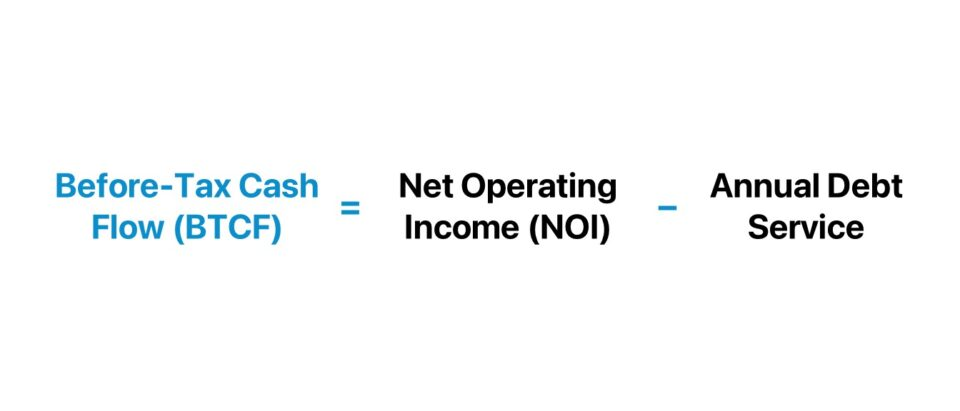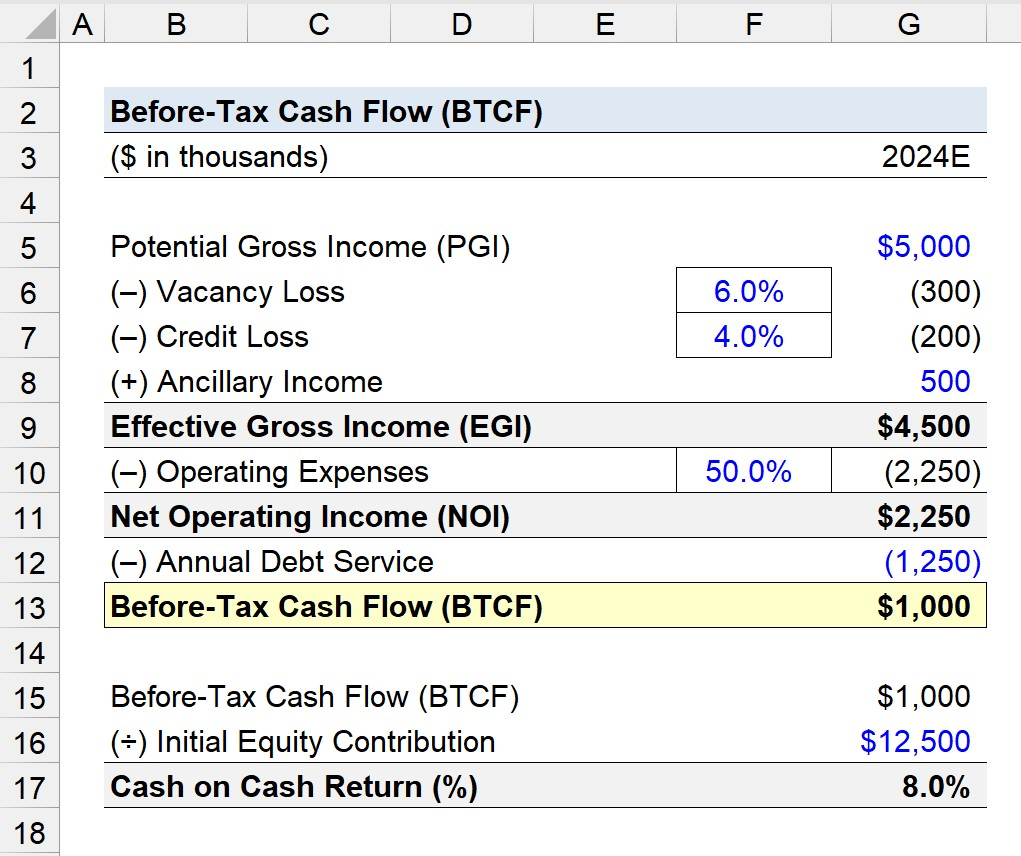Welcome to Wall Street Prep! Use code at checkout for 15% off.# Before-Tax Cash Flow (BTCF)

Guide to Understanding Before-Tax Cash Flow (BTCF)## How to Calculate Before-Tax Cash Flow?

The before-tax cash flow (BTCF) is a real estate metric that measures the remaining pre-tax profit of a rental property, right after meeting its annual debt service.

Therefore, the before-tax cash flow – or “pre-tax cash flow” – is a levered cash flow metric, since the debt costs, such as mortgage payments, are accounted for.

The before-tax cash flow (BTCF) of a property can be determined by subtracting its annual debt service (ADS), such as mortgage payments, from its stabilized net operating income (NOI).

The process of calculating the before-tax cash flow for a given property investment is a four-step process:

1. Determine Potential Gross Income (PGI) → The potential gross income (PGI) is the maximum income that could be earned on the rental property, assuming an occupancy rate of 100%, i.e. no vacant units.
2. Forecast Effective Gross Income (EGI) → From the potential gross income (PGI), subtract vacancy and credit losses, which stem from vacancies (i.e. unoccupied units) and credit losses (i.e. collection issues on rental payments from tenants). The assumptions used must be derived from analyzing market comparables and industry insights relevant to the given property investment.
3. Calculate Net Operating Income (NOI) → The net operating income (NOI) is calculated by subtracting direct property expenses from the effective gross income (EGI). The most common examples of direct operating expenses in real estate include property taxes, property management fees, maintenance costs, and repair fees.
4. Compute Before-Tax Cash Flow (BTCF) → By subtracting the annual debt service from net operating income (NOI), we’re left with the property’s before-tax cash flow. The distinction between NOI and BTCF is that the former is an unlevered metric (“capital structure neutral”), while the latter is a levered metric (“post-financing”).###### Property Taxes in Real Estate

While the before-tax income (BTCF) is above the income tax liability on a real estate pro forma model (and thus, not yet taxed), property taxes are subtracted from BTCF because those costs represent operating items in the real estate sector.

## Before-Tax Cash Flow Formula (BTCF)

The formula to calculate the before-tax cash flow (BTCF) is the difference between the net operating income (NOI) of a property and the annual debt service.

Before-Tax Cash Flow (BTCF) = Net Operating Income (NOI)  Annual Debt Service (ADS)

Where:

• Net Operating Income (NOI) = Effective Gross Income (EGI) – Direct Property Expenses
• Annual Debt Service (ADS) = Principal + Interest

In practice, one of the main use cases of the BTCF metric is to serve as the cash flow metric to calculate the cash on cash return.

The cash on cash return, or “cash yield”, is calculated as the ratio between the before-tax cash flow and initial equity contribution.

Cash on Cash Return (%) = Annual Before-Tax Cash Flow ÷ Equity Contribution

The cash on cash return is thus the yield earned on the original cash contribution paid by the real estate investor, i.e. the down payment.

## Before-Tax Cash Flow Calculator

We’ll now move to a modeling exercise, which you can access by filling out the form below.#### Get the Excel Template!Submitting...

## Before-Tax Cash Flow Calculation Example (BTCF)

Suppose we’re tasked with calculating the before-tax cash flow (BTCF) of a commercial property in 2024.

Year 1 Pro Forma (2024E)

• Potential Gross Income (PGI) = \$5 million
• Vacancy Loss (6.0% of PGI) = \$300k
• Credit Loss (4.0% of PGI) = \$200k
• Ancillary Income = \$500k

Therefore, the effective gross income (EGI) of the property in 2024 is expected to be \$4.5 million.

• Effective Gross Income (EGI) = \$5 million – \$300k – \$200k + \$500k = \$4.5 million

From the effective gross income (EGI), we’ll subtract operating expenses, which we’ll assume to be 50% of EGI, resulting in a net operating income (NOI) of \$2.25 million.

• Net Operating Income (NOI) = \$2.25 million = \$1.25 million = \$1 million

The before-tax cash flow (BTCF) can be determined by deducting the annual debt service (ADS), which we’ll assume to be \$1.25 million, from the stabilized NOI.

Given the net operating income (NOI) at stabilization and annual debt service (ADS), the difference is \$1 million, which represents the property’s before-tax cash flow (BTCF).

• Before-Tax Cash Flow (BTCF) = \$2.25 million – \$1.25 million = \$1 million

In conclusion, we’ll wrap up our quick exercise in Excel by estimating the cash-on-cash return on the real estate investor’s equity investment.

If we assume that the initial equity contribution was \$12.5 million, the implied cash-on-cash return is 8.0%.

• Cash on Cash Return (%) = \$1 million ÷ \$12.5 million = 8.0%20+ Hours of Online Video Training

### Master Real Estate Financial Modeling

This program breaks down everything you need to build and interpret real estate finance models. Used at the world's leading real estate private equity firms and academic institutions.

Inline Feedbacks